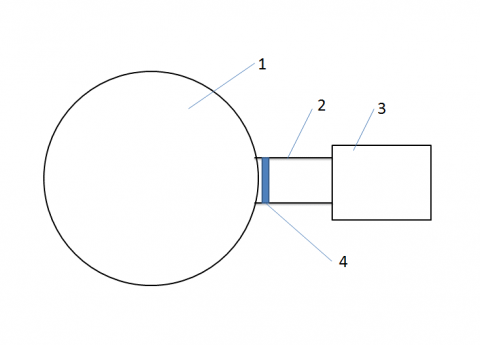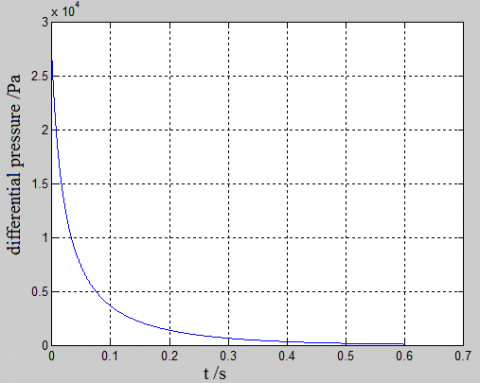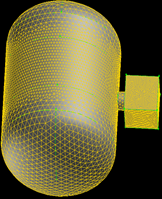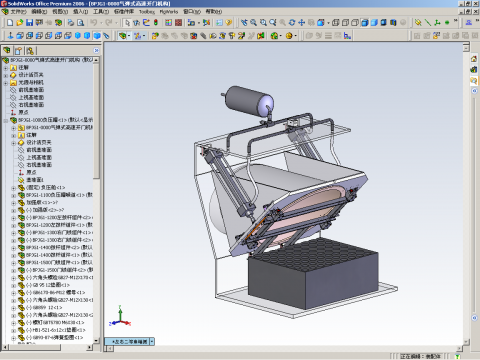# Study on the Decompression Time of the Hypobaric Rapid Decompression Chamber

Study on the Decompression Time of the Hypobaric Rapid Decompression Chamber

Jianfeng Lu* Lin Li Lunjun Chen

College of Mechanical Engineering, Guizhou University, Guiyang, 550025, China

Corresponding Author Email:
cme.jflu@gzu.edu.cn
Page:
75-78
|
DOI:
https://doi.org/10.18280/ijht.330212
N/A
|
Accepted:
N/A
|
Published:
30 June 2015
| Citation

OPEN ACCESS

Abstract:

In order to calculate the decompression time of the hypobaric rapid decompression chamber and obtain the reasonable volume parameter, the equilibrium equation of airflow is deduced and reveals the relationship among the time of barometric pressure balance and pipeline conductance, initial pressure, initial pressure difference and chamber volume. With software FLUENT to make a dynamic simulation on the airflow balance process of decompression chamber during the decompressing. Compared with the experiment datum and calculating results of the two methods, it shows the validity of the two models.

Keywords:

Hypobaric rapid decompression; Pressure balance; Vacuum reserve; Equilibrium equation; Finite element.

1. Introduction

In the atmospheric environment, the hypobaric pressure rapid decompression chamber can achieve the process of rapid decompression from an initial height such as the altitude of 8000 m to a target height 20000-30000m within 100-250ms. So, the process of sudden breakdown and rapid decompression of the aero craft at high altitudes is simulated, the equipment of the aero craft can be tested in the chamber .

Due to the vacuum pump couldn't achieve such rapid decompression, at present, the only way is to use a huge chamber to reserve the vacuum. The vacuum reserve chamber and decompression chamber are connected with a pipe. Firstly, it makes the two chambers reach the preset different vacuum level with the vacuum pump. Then, the valve on the pipe quickly opens, and the rapid decompressing is achieved .

In order to obtain reasonable volume parameters of pipe and chamber, and meet the balanced requirement 100-250ms between two chambers, it needs to calculate the decompression time by the equilibrium equation of airflow and simulate the rapid decompression process of transient airflow.

2. Study on the Airflow Equilibrium Equation of the Decompression Chamber

The physical model of the hypobaric decompression chamber system is shown in figure 1.

## 1.pngFigure 1. Schematic diagram of the hypobaric decompression chamber

Part 1 is the vacuum reserve chamber. Part 2 is the pipe that connects the two chambers. Part 3 is the rapid decompression chamber. They are connected through the pipe, and the valve 4 controls on-off situation between the two chambers. Before decompression, the valve is closed. The absolute pressure of vacuum reserve chamber and decompression chamber are up to about 2000Pa and 30000 Pa by vacuum pump. When the valve is opened quickly, airflow moves rapidly from the high-pressure decompression chamber to the low-pressure vacuum reserve chamber. Then the pressure difference decrease rapidly and eventually reaches balance .

There is the inertial force when the gas molecules flow through the pipe at the pressure balance. Firstly, the airflow is turbulent state, then the inertial force becomes weaker with gradually decreasing pressure difference. Friction plays a major role in the internal gas. At the same time, the airflow changes into the viscous flow state .

It is assumed that pipeline conductance is constant, the expression between two chambers pressure difference and time were derived as follows .

$t=\frac{\ln \frac{\Delta P_{0}}{\Delta P_{t}}}{U\left(\frac{1}{V_{H}}+\frac{1}{V_{L}}\right)}$

The pipeline conductance U is a fixed value in the formulas. In the flow process, the ever-changing flow pattern, outlet pressure and inlet pressure cause the changing pipeline conductance, not a fixed value. In order to calculate the decompression time with the formula, the experiments must be performed to determine the average value of the pipeline conductance first .

It is hard to finish the trials, because of enormous volume of the two chambers and expensive construction costs. It can be assumed that the airflow state is viscous or turbulent, and the corresponding equation is derived. Although there are some errors, it is still referential value for the system design.

Based on the thermodynamic equation of state and the fluid conductance concept, it makes an assumption that airflow is viscous, and the equation of chamber pressure is derived during the decompression process.

The gas is considered as ideal gas in the chamber. Due to short decompression time, it can be assumed that the process is adiabatic and the initial temperature T is invariable in the chamber .

The volume and initial pressure of vacuum chamber are VLand pL0; the volume and initial pressure of decompression chamber are VHand pH0; the pressure of vacuum and decompression chamber are pHtand pLt at the t moment. The balanced time is t. The initial pressure difference and time difference are Δp0= pH0pL0 (pH0> pL0 )andΔpt= pHtpLt.

Based on ideal gas state equation ,

$V_{H} d p_{H t}=R T d m_{H t}$    (1)

$V_{L} d p_{L t}=R T d m_{L_{L}}$       (2)

Because of  $m_{H t}+m_{L t}=C$

$d m_{L t}=-d m_{H t}$       (3)

Combined with the above formulae (1), (2), (3),

$d p_{H t}=\frac{V_{L}}{V_{H}+V_{L}} d \Delta p_{t}$     (4)

$d p_{L t}=\frac{V_{H}}{V_{H}+V_{L}} d \Delta p_{t}$          (5)

Formulae（6）and（7）are  the integration of formulae（4）and（5）

$p_{H t}=\frac{V_{L}}{V_{H}+V_{L}}\left(\Delta p_{t}-\Delta p_{t 0}\right)+p_{H}$        (6)

$p_{L t}=\frac{V_{H}}{V_{H}+V_{L}}\left(\Delta p_{t}-\Delta p_{t 0}\right)+p_{L 0}$   (7)

Formulae (8) is vacuum pumping formulae of vacuum chamber ,

$V_{H} \frac{d p_{H t}}{d t}=-Q$     (8)

Where Q is the flow through the pipe,

$Q=U \cdot \Delta p_{t}$

$=\frac{\pi D^{4}}{128 \eta L} \cdot \frac{p_{H t}+p_{L t}}{2} \Delta p_{t}$           (9)

Where U is the viscous flow conductance of the gas through the pipe, D is the diameter of the pipe, L is the length of the pipe, η is the viscosity of the gas .

Combined with the above formulae (6), (7), (8), (9), the expression of decompression time of the hypobaric rapid decompression chamber can be get as follow.

$t=\frac{\ln \frac{\Delta p_{0}\left(\Delta p_{t}+2 p_{L 0}\right)}{\Delta p_{t}\left(\Delta p_{0}+2 p_{L 0}\right)}}{\frac{\pi D}{128 \eta L}\left(\frac{1}{V_{H}}+\frac{1}{V_{L}}\right) p_{L 0}}$     (10)

Where, the law of the two chambers pressure difference over time is shown in formula (10). At some point, it is relevant among the pressure difference, volume, initial pressure and the geometric dimensioning of pipe. Since the decompression chamber needs to meet the demand that the initial parameters should be ensured in the two chambers.  Enlarging the volume of the vacuum chamber could obtain the balanced time. So the larger conductance connective pipe should be designed and applied. It also can reach the function by enlarging the volume of vacuum chamber. In conclusion, it is necessary to make a reasonable analysis of the connective structure for achieving a specific balance time index. Balanced time can be calculated by formula (10).

For example, The initial pressure and volume of decompression chamber are pH0=2.853×104Pa and VH=9.66m3; The initial pressure and volume of vacuum chamber are pL0=2.15 ×103Pa  and VB=380 m3；The sectional dimension and length of connective pipe are Φ1.0m and 0.5m. The initial temperatures of both chambers are T= 288K. It can obtain the curve of the pressure difference from formula (10). The curve is shown in figure 2. At the beginning of decompression process pressure difference changes rapidly. Then it changes slowly as time goes by.

## 2.pngFigure 2. Differential pressure with time

At the assumption that pressure balance is reached when the pressure difference is less than 500 Pa, the balance time is 336ms .

3. Finite Element Analysis

In order to obtain the accurate results, the FLUNET which is a commercial CFD software is used to simulate the pressure changes.

3.1 Meshing

The size of decompression chamber is 2m*2.3m*2.1m the effective volume of which is 9.66m3. The section size of connection pipe is Φ1.0m whose length is 0.5m. The size of vacuum reserve chamber is Φ8m*10m the effective volume of which is 380m3. Gas is used as Fluid medium. Turbulence is chosen as the flow in the pipe. The meshes is created by using Cooper method when take advantage of the GAMBIT. The finite element model shown in fig has a total number of 64793 nodes and 353239 elements. The unsteady option and the energy equation is chosen in solver, the k-εmodel is selected in the turbulence options .

## 3.pngFigure 3. Meshing

3.2 Boundary conditions

No-slip condition is used on the pipe inner surface. Standard discrete difference is used on the pressure difference. The second-order upwind difference is used on momentum equation, kinetic energy equation and kinetic energy dissipation rate equation. SIMPLE algorithm is taken to solve the pressure equation. The time step is set to 0.002s .

Table1. Boundary conditions setting

 Boundary conditions Setting pressure of vacuum reserve chamber 2150 Pa (26000 meters altitude) pressure of decompression chamber 28530 Pa (9500 meters altitude) temperature 288k wall no-slip

3.3 Simulation Result

## 4.pngFigure 4. Pressure on midpoint of the decompression chamber across the pipe

The simulation result indicates that the pressure of the decompression chamber reaches the balance pressure and begins shocking after 112ms, pressure changes slowly after the first shocking wave.

4. Validation

4.1 Introduction of equipment

Systematic principle of decompression chamber which is consist of decompression chamber, vacuum reserve chamber, vacuum pump system and valve which is shown in fig.5.

## 5.pngFigure 5. Schematic diagram of the equipment

To obtain the ideal decompression time, the valve installed on the connection pipeline of decompression chamber must open quickly. Since the pipe diameter is large, using general type valve can’t meet the requirements of the time, a special valve designed as shown in fig.6 in which the valve opened or closed by air cylinder is tilt installed on the end of pipe at 45 degree. The advantage of it is that the flow area is larger and airflow resistance is smaller when the door opens, simultaneously, it speeds up the open time of value by utilizing the weight of the door, the gas pressure differential between decompression chamber and vacuum reserve chamber as well as the thrust of cylinder, which makes the value open rapidly, so as to shorten the balance time.

## 6.pngFigure 6. Quick opened valve

4.2 Experimental results

High precision data acquisition card of NI Corporation and high frequency absolute pressure sensor of KELLER Corporation is used to collect data. The curve of pressure in the process of decompression is shown in fig.7.

## 7.pngFigure 7. Collceted experimental curve of the pressure in decompression chamber

The experimental result indicates that the pressure of the decompression chamber reaches the balance pressure and begins shocking after 127ms, pressure changes slowly after the first shocking wave.

4.3 Comparison and analysis

The laws of change of the decompression chamber pressure with time is obtained by using mathematical method, the finite element simulation and the experiment, the comparison of data and curve is shown in table.1.The curve and data obtained by finite element simulation is close to the experiment data, which shows that the precision of the finite element analysis is better than that of mathematical equations.

The fluctuation appears in finite element simulation as well as the experiment when the pressure balance is reached. Since the rapid flow of the gas produced a shock wave, the high pressure appears in which the wave arrive. After the shock wave leave, the pressure reduced. Because the gas continues to flow, it reduces to below the pressure when reached the pressure balance. Due to the repeated oscillation of the airflow in the chamber with finite volume, the fluctuation appears in the experiment when the pressure reduced to below the balance.

Since the valve’s open time which is longer than 0 second in fact is set to 0 second in the finites element simulation and mathematical equations, the falling speed of incipient stage in curves of experiment is slower than that of finite element simulation and mathematical equations. According to the table.2, the higher pressure the cylinder has, the shorter time of opening the valve will get as well as the decompression time of experiment.

Table 2. Data comparison

 Vacuum reserve chamber (Pa) Decompression chamber’s pressure(Pa) Cylinder pressure (MPa) Decompression time Mathematical method(ms) FEM(ms) Experiment(ms) 2150 28530 1.2 336 112 127 0.8 136
5. Conclusions

(1) Mathematical expression of decompression based on viscous flow can reveal the relationship between the difference pressure between tow chamber and the initial conditions, the connection pipeline and the decompression time, which can be estimate the decompression time roughly.

(2) The process of pressure reaching to the balance is simulated by utilizing the FLUNET software. The curve obtained by finite element analysis can be used to get the precise time required to reach the balance pressure of two chambers.

(3) Because of the action delay of valve and other equipment, the decompression time of experiment is longer than that of the finite element simulation. In general, the results of computation agree well with that of experiment, which prove the correctness of the finite element model.

Acknowledgement

The study is supported by Foundation of Guizhou Science and Technology Department (No. 2121 and 6002).

References

1. Yin Dong-chen, Xiao Hua-jun, Zang Bin. Development of Experiment Chambers of Complex L ow -pressure Environment [J]. Chinese Medical Equipment Journal, 2011, 32 (12): 6-8.

2. Matuska, John H., Taulbee, Carl D. Hypobaric test chamber design [J]. American Society of Mechanical Engineers, Pressure Vessels and Piping Division (Publication) PVP, 2000, 401: 151-157.

3. Jan Ove Owe, John Horthe. Hypobaric chamber operations at the Institute of Aviation Medicine, Oslo. Norway[R]. Norway: NAMA, 2005: 9-10.

4. Emael M. Rapid Decompression Emergencies in Pressure Suited Subjects [J]. Air Medical Journal, 2007, 28 (1): 82 – 91.

5. Eoffrey A. Mathematical Model Research of Human Exposure to Vacuum [J]. Journal of the British Interplanetary Society, 2008, 29 (2): 148 – 154.

6. Chen Shuping, Da Daoan, Chen Guangqi, Analysis of Pressure Balancing Time in Connecting Two Chambers of Space Station [J]. Chinese Journal of Vacuum Science and Technology, 2010, 3 0 (3): 226-228.

7. Demtrad. On the Decompression of a Punctured Pressu – rized Cabin in Vacuum Flight [J]. Jet Propulsion, 2006, 24 (2): 112 – 123.

8. Ding Zhu-rong. Fluid Mechanics [M].Beijing: Higher Education Press, 2003:12-14.

9. Crane co. Flow of Fluids Through Valves, Fittings and Pipe: Technical Paper No.410M [P]. Engineering Department, Crane co.2009:33-34.

10. Smith J M, Van H C, Abbot M M. Introduction to Chemical Engineering Thermodynamics [M]. Beijing: Chemical Industry Press, 2002: 62-78.

11. Li Jun-Jian, Wang Xiao-J. Vacuum Technology [M]. BeiJing: National Defence of Industry Press, 2014: 76-77.

12. Coelho, P.M and Pinho. Considerations About Equations for Steady State Flow in Nature Gas Pipelines [J]. Journal of the Brazilian Society of Mechanical Sciences & Engineering, 2007, 29(3): 262-273.

13. Bréard, Cyrille, Lednicer, David, Lachendro, Nate, Murvine, Everett. A CFD analysis of sudden cockpit decompression [C]. AIAA Paper, 2004: 4001-4010.

14. Patro, Brundaban, Murugan, S., Patro, Pandaba. Numerical modeling of gas-solid flow in a horizontal pipe [J]. Multiphase Science and Technology, 2012, 24(4): 299-322.

15. Hou-Qingmin, Jiao-Wenling. Improved FDS analysis for the atmospheric impact of natural gas leakage and diffusion [J]. Journal of Computational Information Systems, 2011, 7(13): 4702-4709.